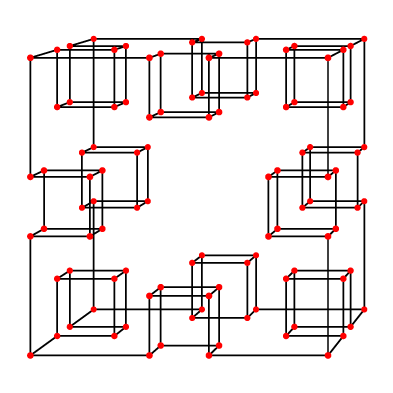I was thinking again today about a minor point related to my SoCG 2010 paper with Elena Mumford on orthogonal polyhedra (polyhedra with the topology of the sphere, and with each vertex having three edges parallel to the three coordinate axes). In that paper we characterized the graphs of these polyhedra as being the 3-regular planar bipartite graphs satisfying two connectivity conditions: they are biconnected (removing any single vertex cannot make more than one connected component), and additionally removing any two vertices cannot make more than two connected components.

It reminded me a bit of graph toughness: a graph is 1-tough if removing any set of vertices cannot make more connected components than the number of removed vertices. And more generally, a graph is t-tough if, no matter how you remove some set S of vertices, the remaining subgraph doesn't ever have more than t|S| components. It made me wonder: maybe there is a simpler way of stating our characterization using toughness?

The answer is no. The orthogonal polyhedron below (formed by putting a small cubical dent in each of the twelve edges of a large cube) is only 2/3-tough: remove the eight outer corner vertices and the remaining graph falls into twelve pieces. And on the other hand there are 3-regular planar bipartite graphs that are 2/3-tough but are not the graphs of orthogonal polyhedra.However, except for borderline cases such as this, toughness can be used as a substitute for the connectivity conditions: if a graph is $$t$$-tough for any $$t$$ greater than 2/3 (and is 3-regular, bipartite, and planar), then it is the graph of an orthogonal polyhedron, because the two connectivity conditions follow immediately from toughness. And in the other direction every orthogonal polyhedron (and more generally every biconnected 3-regular graph) is at least 2/3-tough, by an easy double counting argument on the number of incidences of components to cut vertices.

Incidentally the same example shows that orthogonal polyhedra are not always Hamiltonian, so Barnette's conjecture on the Hamiltonicity of 3-connected 3-regular bipartite planar graphs does not generalize to orthogonal polyhedra.